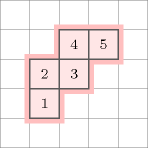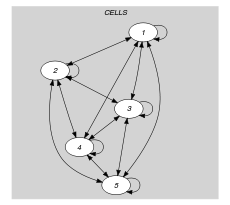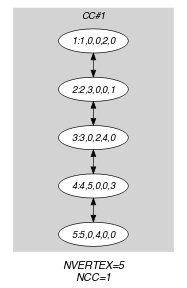## 5.324. polyomino

Origin

Inspired by [Golomb65].

Constraint

$\mathrm{𝚙𝚘𝚕𝚢𝚘𝚖𝚒𝚗𝚘}\left(\mathrm{𝙲𝙴𝙻𝙻𝚂}\right)$

Argument
 $\mathrm{𝙲𝙴𝙻𝙻𝚂}$ $\mathrm{𝚌𝚘𝚕𝚕𝚎𝚌𝚝𝚒𝚘𝚗}\left(\begin{array}{c}\mathrm{𝚒𝚗𝚍𝚎𝚡}-\mathrm{𝚒𝚗𝚝},\hfill \\ \mathrm{𝚛𝚒𝚐𝚑𝚝}-\mathrm{𝚍𝚟𝚊𝚛},\hfill \\ \mathrm{𝚕𝚎𝚏𝚝}-\mathrm{𝚍𝚟𝚊𝚛},\hfill \\ \mathrm{𝚞𝚙}-\mathrm{𝚍𝚟𝚊𝚛},\hfill \\ \mathrm{𝚍𝚘𝚠𝚗}-\mathrm{𝚍𝚟𝚊𝚛}\hfill \end{array}\right)$
Restrictions
 $\mathrm{𝙲𝙴𝙻𝙻𝚂}.\mathrm{𝚒𝚗𝚍𝚎𝚡}\ge 1$ $\mathrm{𝙲𝙴𝙻𝙻𝚂}.\mathrm{𝚒𝚗𝚍𝚎𝚡}\le |\mathrm{𝙲𝙴𝙻𝙻𝚂}|$ $|\mathrm{𝙲𝙴𝙻𝙻𝚂}|\ge 1$ $\mathrm{𝚛𝚎𝚚𝚞𝚒𝚛𝚎𝚍}$$\left(\mathrm{𝙲𝙴𝙻𝙻𝚂},\left[\mathrm{𝚒𝚗𝚍𝚎𝚡},\mathrm{𝚛𝚒𝚐𝚑𝚝},\mathrm{𝚕𝚎𝚏𝚝},\mathrm{𝚞𝚙},\mathrm{𝚍𝚘𝚠𝚗}\right]\right)$ $\mathrm{𝚍𝚒𝚜𝚝𝚒𝚗𝚌𝚝}$$\left(\mathrm{𝙲𝙴𝙻𝙻𝚂},\mathrm{𝚒𝚗𝚍𝚎𝚡}\right)$ $\mathrm{𝙲𝙴𝙻𝙻𝚂}.\mathrm{𝚛𝚒𝚐𝚑𝚝}\ge 0$ $\mathrm{𝙲𝙴𝙻𝙻𝚂}.\mathrm{𝚛𝚒𝚐𝚑𝚝}\le |\mathrm{𝙲𝙴𝙻𝙻𝚂}|$ $\mathrm{𝙲𝙴𝙻𝙻𝚂}.\mathrm{𝚕𝚎𝚏𝚝}\ge 0$ $\mathrm{𝙲𝙴𝙻𝙻𝚂}.\mathrm{𝚕𝚎𝚏𝚝}\le |\mathrm{𝙲𝙴𝙻𝙻𝚂}|$ $\mathrm{𝙲𝙴𝙻𝙻𝚂}.\mathrm{𝚞𝚙}\ge 0$ $\mathrm{𝙲𝙴𝙻𝙻𝚂}.\mathrm{𝚞𝚙}\le |\mathrm{𝙲𝙴𝙻𝙻𝚂}|$ $\mathrm{𝙲𝙴𝙻𝙻𝚂}.\mathrm{𝚍𝚘𝚠𝚗}\ge 0$ $\mathrm{𝙲𝙴𝙻𝙻𝚂}.\mathrm{𝚍𝚘𝚠𝚗}\le |\mathrm{𝙲𝙴𝙻𝙻𝚂}|$
Purpose

Enforce all cells of the collection $\mathrm{𝙲𝙴𝙻𝙻𝚂}$ to be connected and to form a single block. Each cell is defined by the following attributes:

1. The $\mathrm{𝚒𝚗𝚍𝚎𝚡}$ attribute of the cell, which is an integer between 1 and the total number of cells, is unique for each cell.

2. The $\mathrm{𝚛𝚒𝚐𝚑𝚝}$ attribute that is the index of the cell located immediately to the right of that cell (or 0 if no such cell exists).

3. The $\mathrm{𝚕𝚎𝚏𝚝}$ attribute that is the index of the cell located immediately to the left of that cell (or 0 if no such cell exists).

4. The $\mathrm{𝚞𝚙}$ attribute that is the index of the cell located immediately on top of that cell (or 0 if no such cell exists).

5. The $\mathrm{𝚍𝚘𝚠𝚗}$ attribute that is the index of the cell located immediately above that cell (or 0 if no such cell exists).

This corresponds to a polyomino [Golomb65].

Example
$\left(\begin{array}{c}〈\begin{array}{ccccc}\mathrm{𝚒𝚗𝚍𝚎𝚡}-1\hfill & \mathrm{𝚛𝚒𝚐𝚑𝚝}-0\hfill & \mathrm{𝚕𝚎𝚏𝚝}-0\hfill & \mathrm{𝚞𝚙}-2\hfill & \mathrm{𝚍𝚘𝚠𝚗}-0,\hfill \\ \mathrm{𝚒𝚗𝚍𝚎𝚡}-2\hfill & \mathrm{𝚛𝚒𝚐𝚑𝚝}-3\hfill & \mathrm{𝚕𝚎𝚏𝚝}-0\hfill & \mathrm{𝚞𝚙}-0\hfill & \mathrm{𝚍𝚘𝚠𝚗}-1,\hfill \\ \mathrm{𝚒𝚗𝚍𝚎𝚡}-3\hfill & \mathrm{𝚛𝚒𝚐𝚑𝚝}-0\hfill & \mathrm{𝚕𝚎𝚏𝚝}-2\hfill & \mathrm{𝚞𝚙}-4\hfill & \mathrm{𝚍𝚘𝚠𝚗}-0,\hfill \\ \mathrm{𝚒𝚗𝚍𝚎𝚡}-4\hfill & \mathrm{𝚛𝚒𝚐𝚑𝚝}-5\hfill & \mathrm{𝚕𝚎𝚏𝚝}-0\hfill & \mathrm{𝚞𝚙}-0\hfill & \mathrm{𝚍𝚘𝚠𝚗}-3,\hfill \\ \mathrm{𝚒𝚗𝚍𝚎𝚡}-5\hfill & \mathrm{𝚛𝚒𝚐𝚑𝚝}-0\hfill & \mathrm{𝚕𝚎𝚏𝚝}-4\hfill & \mathrm{𝚞𝚙}-0\hfill & \mathrm{𝚍𝚘𝚠𝚗}-0\hfill \end{array}〉\hfill \end{array}\right)$

The $\mathrm{𝚙𝚘𝚕𝚢𝚘𝚖𝚒𝚗𝚘}$ constraint holds since all the cells corresponding to the items of the $\mathrm{𝙲𝙴𝙻𝙻𝚂}$ collection form one single group of connected cells: the ${i}^{th}$ ($i\in \left[1,4\right]$) cell is connected to the ${\left(i+1\right)}^{th}$ cell. Figure 5.324.1 shows the corresponding polyomino.

##### Figure 5.324.1. Polyomino corresponding to the Example slot where each cell contains the index of the corresponding item within the $\mathrm{𝙲𝙴𝙻𝙻𝚂}$ collectionSymmetries
• Items of $\mathrm{𝙲𝙴𝙻𝙻𝚂}$ are permutable.

• Attributes of $\mathrm{𝙲𝙴𝙻𝙻𝚂}$ are permutable w.r.t. permutation $\left(\mathrm{𝚒𝚗𝚍𝚎𝚡}\right)$ $\left(\mathrm{𝚛𝚒𝚐𝚑𝚝},\mathrm{𝚕𝚎𝚏𝚝}\right)$ $\left(\mathrm{𝚞𝚙}\right)$ $\left(\mathrm{𝚍𝚘𝚠𝚗}\right)$ (permutation applied to all items).

• Attributes of $\mathrm{𝙲𝙴𝙻𝙻𝚂}$ are permutable w.r.t. permutation $\left(\mathrm{𝚒𝚗𝚍𝚎𝚡}\right)$ $\left(\mathrm{𝚛𝚒𝚐𝚑𝚝}\right)$ $\left(\mathrm{𝚕𝚎𝚏𝚝}\right)$ $\left(\mathrm{𝚞𝚙},\mathrm{𝚍𝚘𝚠𝚗}\right)$ (permutation applied to all items).

• Attributes of $\mathrm{𝙲𝙴𝙻𝙻𝚂}$ are permutable w.r.t. permutation $\left(\mathrm{𝚒𝚗𝚍𝚎𝚡}\right)$ $\left(\mathrm{𝚞𝚙},\mathrm{𝚕𝚎𝚏𝚝},\mathrm{𝚍𝚘𝚠𝚗},\mathrm{𝚛𝚒𝚐𝚑𝚝}\right)$ (permutation applied to all items).

Usage

Enumeration of polyominoes.

Keywords
Arc input(s)

$\mathrm{𝙲𝙴𝙻𝙻𝚂}$

Arc generator
$\mathrm{𝐶𝐿𝐼𝑄𝑈𝐸}$$\left(\ne \right)↦\mathrm{𝚌𝚘𝚕𝚕𝚎𝚌𝚝𝚒𝚘𝚗}\left(\mathrm{𝚌𝚎𝚕𝚕𝚜}\mathtt{1},\mathrm{𝚌𝚎𝚕𝚕𝚜}\mathtt{2}\right)$

Arc arity
Arc constraint(s)
$\bigvee \left(\begin{array}{c}\bigwedge \left(\begin{array}{c}\mathrm{𝚌𝚎𝚕𝚕𝚜}\mathtt{1}.\mathrm{𝚛𝚒𝚐𝚑𝚝}=\mathrm{𝚌𝚎𝚕𝚕𝚜}\mathtt{2}.\mathrm{𝚒𝚗𝚍𝚎𝚡},\hfill \\ \mathrm{𝚌𝚎𝚕𝚕𝚜}\mathtt{2}.\mathrm{𝚕𝚎𝚏𝚝}=\mathrm{𝚌𝚎𝚕𝚕𝚜}\mathtt{1}.\mathrm{𝚒𝚗𝚍𝚎𝚡}\hfill \end{array}\right),\hfill \\ \bigwedge \left(\begin{array}{c}\mathrm{𝚌𝚎𝚕𝚕𝚜}\mathtt{1}.\mathrm{𝚕𝚎𝚏𝚝}=\mathrm{𝚌𝚎𝚕𝚕𝚜}\mathtt{2}.\mathrm{𝚒𝚗𝚍𝚎𝚡},\hfill \\ \mathrm{𝚌𝚎𝚕𝚕𝚜}\mathtt{2}.\mathrm{𝚛𝚒𝚐𝚑𝚝}=\mathrm{𝚌𝚎𝚕𝚕𝚜}\mathtt{1}.\mathrm{𝚒𝚗𝚍𝚎𝚡}\hfill \end{array}\right),\hfill \\ \mathrm{𝚌𝚎𝚕𝚕𝚜}\mathtt{1}.\mathrm{𝚞𝚙}=\mathrm{𝚌𝚎𝚕𝚕𝚜}\mathtt{2}.\mathrm{𝚒𝚗𝚍𝚎𝚡}\wedge \mathrm{𝚌𝚎𝚕𝚕𝚜}\mathtt{2}.\mathrm{𝚍𝚘𝚠𝚗}=\mathrm{𝚌𝚎𝚕𝚕𝚜}\mathtt{1}.\mathrm{𝚒𝚗𝚍𝚎𝚡},\hfill \\ \mathrm{𝚌𝚎𝚕𝚕𝚜}\mathtt{1}.\mathrm{𝚍𝚘𝚠𝚗}=\mathrm{𝚌𝚎𝚕𝚕𝚜}\mathtt{2}.\mathrm{𝚒𝚗𝚍𝚎𝚡}\wedge \mathrm{𝚌𝚎𝚕𝚕𝚜}\mathtt{2}.\mathrm{𝚞𝚙}=\mathrm{𝚌𝚎𝚕𝚕𝚜}\mathtt{1}.\mathrm{𝚒𝚗𝚍𝚎𝚡}\hfill \end{array}\right)$
Graph property(ies)
 $•$$\mathrm{𝐍𝐕𝐄𝐑𝐓𝐄𝐗}$$=|\mathrm{𝙲𝙴𝙻𝙻𝚂}|$ $•$$\mathrm{𝐍𝐂𝐂}$$=1$

Graph model

The graph constraint models the fact that all the cells are connected. We use the $\mathrm{𝐶𝐿𝐼𝑄𝑈𝐸}\left(\ne \right)$ arc generator in order to only consider connections between two distinct cells. The first graph property $\mathrm{𝐍𝐕𝐄𝐑𝐓𝐄𝐗}=|\mathrm{𝙲𝙴𝙻𝙻𝚂}|$ avoid the case isolated cells, while the second graph property $\mathrm{𝐍𝐂𝐂}=1$ enforces to have a single group of connected cells.

Parts (A) and (B) of Figure 5.324.2 respectively show the initial and final graph associated with the Example slot. Since we use the $\mathrm{𝐍𝐕𝐄𝐑𝐓𝐄𝐗}$ graph property the vertices of the final graph are stressed in bold. Since we also use the $\mathrm{𝐍𝐂𝐂}$ graph property we show the unique connected component of the final graph. An arc between two vertices indicates that two cells are directly connected.

##### Figure 5.324.2. Initial and final graph of the $\mathrm{𝚙𝚘𝚕𝚢𝚘𝚖𝚒𝚗𝚘}$ constraint(a) (b)
Signature

From the graph property $\mathrm{𝐍𝐕𝐄𝐑𝐓𝐄𝐗}=|\mathrm{𝙲𝙴𝙻𝙻𝚂}|$ and from the restriction $|\mathrm{𝙲𝙴𝙻𝙻𝚂}|\ge 1$ we have that the final graph is not empty. Therefore it contains at least one connected component. So we can rewrite $\mathrm{𝐍𝐂𝐂}=1$ to $\mathrm{𝐍𝐂𝐂}\le 1$ and simplify $\underline{\overline{\mathrm{𝐍𝐂𝐂}}}$ to $\underline{\mathrm{𝐍𝐂𝐂}}$.